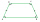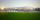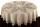# A trapezoid

A trapezoid 75 ft wide on top 85 ft on the bottom, the height is 120 ft. What is its area in the square yds?

Result

S =  1066.667 yd2

#### Solution:Leave us a comment of example and its solution (i.e. if it is still somewhat unclear...):

Showing 0 comments:Be the first to comment!#### To solve this example are needed these knowledge from mathematics:

Do you want to convert area units?

## Next similar examples:

1. Trapezium basesFind the trapezium height if a = 8 cm and c = 4 cm if its content 21 square centimeters.
2. Roof tilesThe roof has a trapezoidal shape with bases of 15 m and 10 m, height of roof is 4 meters. How many tiles will need if on 1 m2 should be used 8 tiles?
3. Trapezium 2Trapezium has an area of 24 square cms. How many different trapeziums can be formed ?
4. TrapeziumThe area of trapezium is 35cm2 find its altitude if the bases are 6cm and 8cm.
5. Area of ditchHow great content area will have a section of trapezoidal ditch with a width of 1.6 meters above and below 0.57 meters? The depth of the ditch is 2.08 meters.
6. Isosceles trapezoid v3In an isosceles trapezoid ABCD is the size of the angle β = 81° Determine size of angles α, γ and δ.
7. HřištěMap scale is 1: 5000. The playground is rectangular and on the map has dimensions 10 cm and 5 cm What is area of playground in square meters in reality?
8. GardenThe garden around the new majer is divided as follows: 35% vegetable, 30% fruit orchards, 10% flowers and the remaining 120 m2 are lawns. What is the total area of the garden?
9. Temperature conversionThe normal human body temperature is 98.6 degrees Fahrenheit. What is the temperature in degrees Celcius?
10. One hectareHow many square meters are one hectare?
11. RapeThe agricultural cooperative harvested 525 ares of rape, of which received 5.6 tons of rape seeds. Calculate the yield per hectare of rape.
12. Round table tableclothRound table has area 50.24 dm2. Calculate the diameter of the circular tablecloth, if it is extend beyond the edge of the table by 30 cm.
13. LandLand has a rectangular shape, its surface area is 1.45 hectares. Its width is 250 m. Determine the length of the land.
14. Pipe cross sectionThe pipe has an outside diameter 1100 mm and the pipe wall is 100 mm thick. Calculate the cross section of this pipe.
15. RhombusPQRS is a rhombus. Given that PQ=3 cm & height of rhombus is given 2 cm. Calculate its area.
16. Feet to milesA student runs 2640 feet. If the student runs an additional 7920 feet, how many total miles does the student run?
17. Degrees to radiansConvert magnitude of the angle α = 9°39'15" to radians: